# Pandas的apply, map, transform介绍和性能测试

apply函数是我们经常用到的一个Pandas操作。虽然这在较小的数据集上不是问题，但在处理大量数据时，由此引起的性能问题会变得更加明显。虽然apply的灵活性使其成为一个简单的选择，但本文介绍了其他Pandas函数作为潜在的替代方案。

`````` df_english=pd.DataFrame(
{
"student": ["John", "James", "Jennifer"],
"gender": ["male", "male", "female"],
"score": [20, 30, 30],
"subject": "english"
}
)

df_math=pd.DataFrame(
{
"student": ["John", "James", "Jennifer"],
"gender": ["male", "male", "female"],
"score": [90, 100, 95],
"subject": "math"
}
)
df=pd.concat(
[df_english, df_math],
ignore_index=True
)``````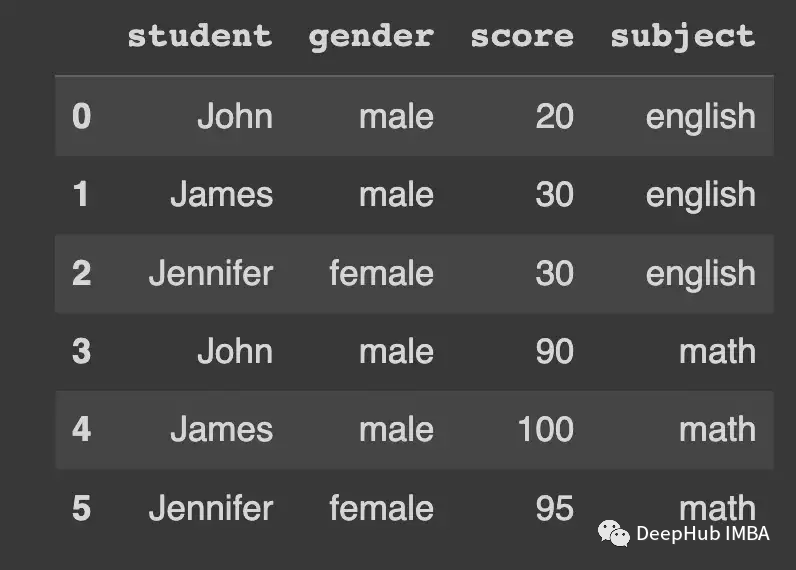## map

`` Series.map(arg, na_action=None) -> Series``

map方法适用于Series，它基于传递给函数的参数将每个值进行映射。arg可以是一个函数——就像apply可以取的一样——也可以是一个字典或一个Series。

na_action是指定序列的NaN值如何处理。当设置为"ignore "时，arg将不会应用于NaN值。

`````` GENDER_ENCODING= {
"male": 0,
"female": 1
}
df["gender"].map(GENDER_ENCODING)``````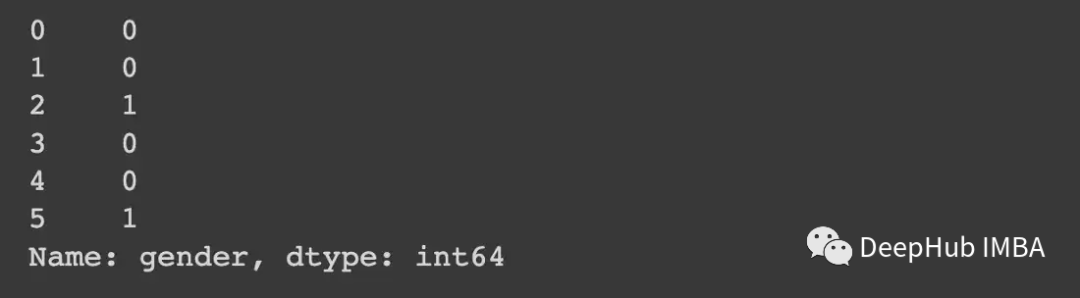`````` df["gender"].apply(lambdax:
GENDER_ENCODING.get(x, np.nan)
)``````

`````` random_gender_series=pd.Series([
random.choice(["male", "female"]) for_inrange(1_000_000)
])

random_gender_series.value_counts()

"""
>>>
female    500094
male      499906
dtype: int64
"""``````

`````` """
map performance
"""
%%timeit
random_gender_series.map(GENDER_ENCODING)

# 41.4 ms ± 4.24 ms per loop (mean ± std. dev. of 7 runs, 10 loops each)

"""
apply performance
"""
%%timeit
random_gender_series.apply(lambdax:
GENDER_ENCODING.get(x, np.nan)
)

# 417 ms ± 5.32 ms per loop (mean ± std. dev. of 7 runs, 1 loop each)``````

## applymap

`` DataFrame.applymap(func, na_action=None, **kwargs) -> DataFrame``

applymap与map非常相似，并且是使用apply内部实现的。applymap就像map一样，但是是在DataFrame上以elementwise的方式工作，但由于它是由apply内部实现的，所以它不能接受字典或Series作为输入——只允许使用函数。

`````` try:
df.applymap(dict())

exceptTypeErrorase:
print("Only callables are valid! Error:", e)

"""
Only callables are valid! Error: the first argument must be callable
"""``````

na_action的工作原理和map中的一样。

## transform

`` DataFrame.transform(func, axis=0, *args, **kwargs) -> DataFrame``

`````` df.groupby("subject")["score"] \
.transform(
lambdax: (x-x.mean()) /x.std()
)

"""
0   -1.154701
1    0.577350
2    0.577350
3   -1.000000
4    1.000000
5    0.000000
Name: score, dtype: float64``````

`````` df.groupby("subject")["score"] \
.apply(
lambdax: (x-x.mean()) /x.std()
)

"""
0   -1.154701
1    0.577350
2    0.577350
3   -1.000000
4    1.000000
5    0.000000
Name: score, dtype: float64
"""``````

Transform必须返回一个与它所应用的轴长度相同的数据框架。

`````` df.groupby("subject")["score"] \
.apply(
sum
)

"""
subject
english     80
math       285
Name: score, dtype: int64
"""``````

`````` df.groupby("subject")["score"] \
.transform(
sum
)

"""
0     80
1     80
2     80
3    285
4    285
5    285
Name: score, dtype: int64
"""``````

`````` try:
df["score"].transform("mean")
exceptValueErrorase:
print("Aggregation doesn't work with transform. Error:", e)

"""
Aggregation doesn't work with transform. Error: Function did not transform
"""``````

`````` df["score"].apply("mean")

"""
60.833333333333336
"""``````

`````` random_score_df=pd.DataFrame({
"subject": random.choices(["english", "math", "science", "history"], k=1_000_000),
"score": random.choices(list(np.arange(1, 100)), k=1_000_000)
})``````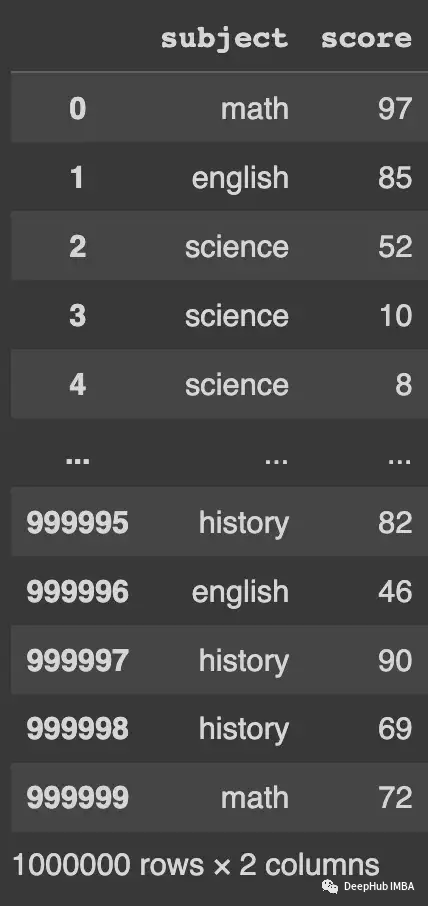`````` """
Transform Performance Test
"""
%%timeit
random_score_df.groupby("subject")["score"] \
.transform(
lambdax: (x-x.mean()) /x.std()
)

"""
202 ms ± 5.37 ms per loop (mean ± std. dev. of 7 runs, 1 loop each)
"""
"""
Apply Performance Test
"""
%%timeit
random_score_df.groupby("subject")["score"] \
.apply(
lambdax: (x-x.mean()) /x.std()
)

"""
401 ms ± 5.37 ms per loop (mean ± std. dev. of 7 runs, 1 loop each)
"""``````

## agg

`````` DataFrame.agg(func=None, axis=0, *args, **kwargs)
-> scalar | pd.Series | pd.DataFrame``````

agg函数更容易理解，因为它只是返回传递给它的数据的聚合。所以无论自定义聚合器是如何实现的，结果都将是传递给它的每一列的单个值。

`` df.groupby("subject")["score"].agg(mean_score="mean").round(2)``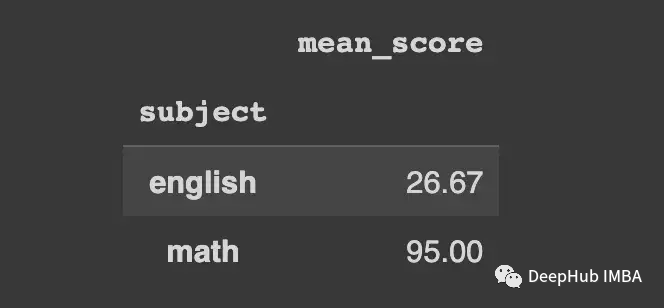`````` df.groupby("subject")["score"].agg(
["min", "mean", "max"]
).round(2)``````Agg提供了更多执行聚合的选项。我们还可以构建自定义聚合器，并对每一列执行多个特定的聚合，例如计算一列的平均值和另一列的中值。

`````` random_score_df=pd.DataFrame({
"subject": random.choices(["english", "math", "science", "history"], k=1_000_000),
"score": random.choices(list(np.arange(1, 100)), k=1_000_000)
})```````````` """
Agg Performance Test
"""

%%timeit
random_score_df.groupby("subject")["score"].agg("mean")

"""
74.2 ms ± 5.02 ms per loop (mean ± std. dev. of 7 runs, 10 loops each)
"""

"""
Apply Performance Test
"""

%%timeit
random_score_df.groupby("subject")["score"].apply(lambda x: x.mean())
"""
102.3 ms ± 1.16 ms per loop (mean ± std. dev. of 7 runs, 10 loops each)
"""``````

`````` """
Multiple Aggregators Performance Test with agg
"""
%%timeit
random_score_df.groupby("subject")["score"].agg(
["min", "mean", "max"]
)

"""
90.5 ms ± 16.7 ms per loop (mean ± std. dev. of 7 runs, 1 loop each)
"""
"""
Multiple Aggregators Performance Test with apply
"""
%%timeit
random_score_df.groupby("subject")["score"].apply(
lambda x: pd.Series(
{"min": x.min(), "mean": x.mean(), "max": x.max()}
)
).unstack()

"""
104 ms ± 5.78 ms per loop (mean ± std. dev. of 7 runs, 10 loops each)
"""``````

## apply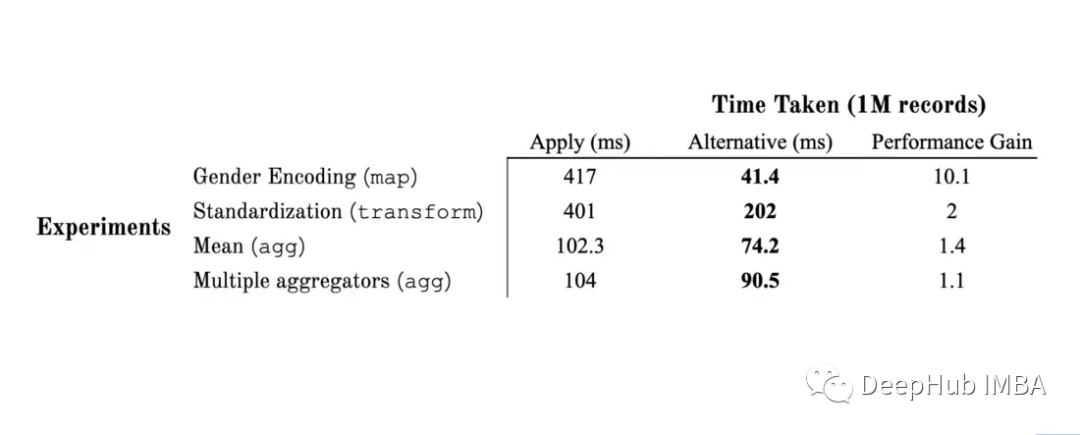## apply的一些问题

apply灵活性是非常好的，但是它也有一些问题，比如

`````` df_single_group=df.copy()
df_single_group["city"] ="Boston"``````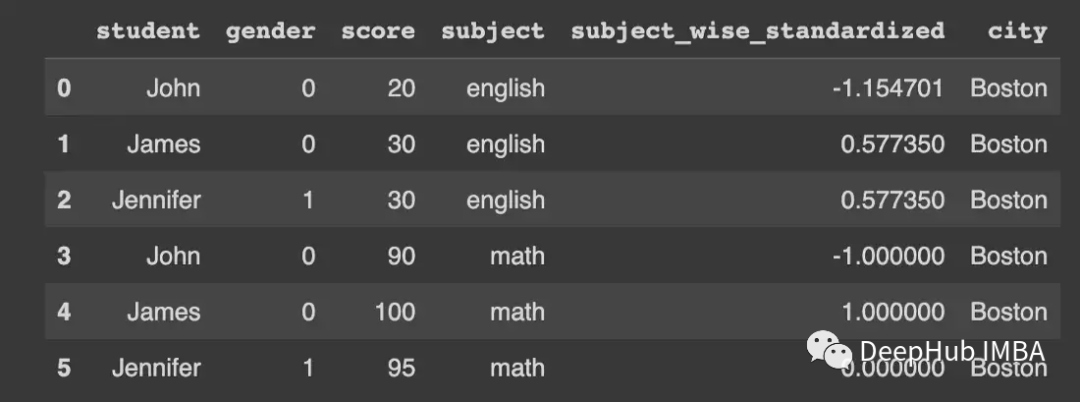`` df_single_group.groupby("subject").apply(lambda x: x["score"])``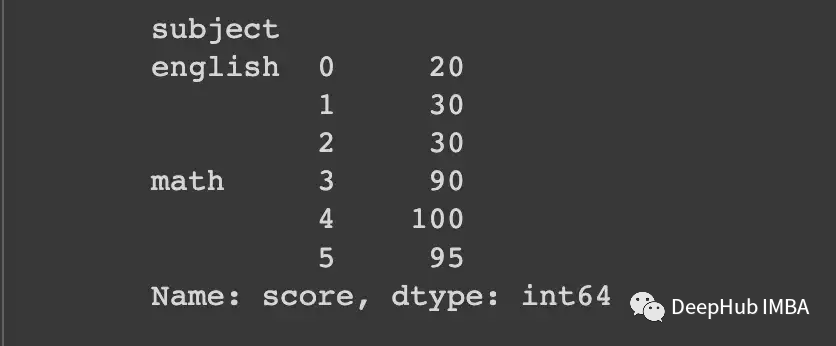`` df_single_group.groupby("city").apply(lambda x: x["score"])```` df_single_group.groupby("city").apply(lambda x: x["score"]).stack()``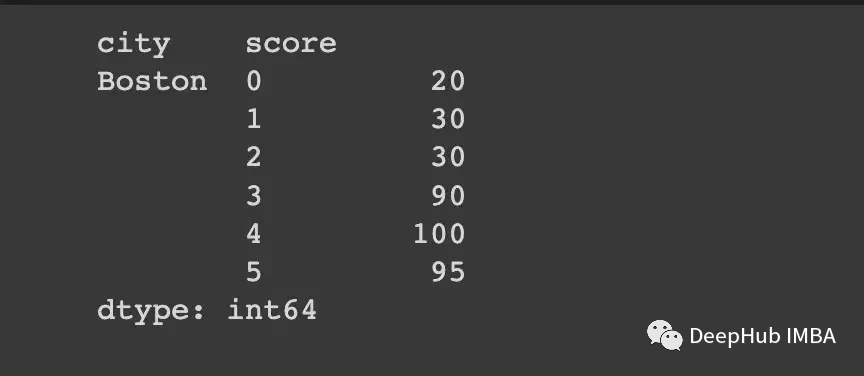## 总结

apply提供的灵活性使其在大多数场景中成为非常方便的选择，所以如果你的数据不大，或者对处理时间没有硬性的要求，那就直接使用apply吧。如果真的对时间有要求，还是找到优化的方式来操作，这样可以省去大量的时间。

1 阅读 121
##### 推荐阅读3959

460
SegmentFault 思否旗下人工智能领域产业媒体，专注技术与产业，一起探索人工智能。